RRB JE CBT 1 - Full Test 2

# RRB JE CBT 1 - Full Test 2

Test Description

## 100 Questions MCQ Test RRB JE (Railways Junior Engineer) CBT Mock Test Series | RRB JE CBT 1 - Full Test 2

RRB JE CBT 1 - Full Test 2 for Railways 2023 is part of RRB JE (Railways Junior Engineer) CBT Mock Test Series preparation. The RRB JE CBT 1 - Full Test 2 questions and answers have been prepared according to the Railways exam syllabus.The RRB JE CBT 1 - Full Test 2 MCQs are made for Railways 2023 Exam. Find important definitions, questions, notes, meanings, examples, exercises, MCQs and online tests for RRB JE CBT 1 - Full Test 2 below.
Solutions of RRB JE CBT 1 - Full Test 2 questions in English are available as part of our RRB JE (Railways Junior Engineer) CBT Mock Test Series for Railways & RRB JE CBT 1 - Full Test 2 solutions in Hindi for RRB JE (Railways Junior Engineer) CBT Mock Test Series course. Download more important topics, notes, lectures and mock test series for Railways Exam by signing up for free. Attempt RRB JE CBT 1 - Full Test 2 | 100 questions in 90 minutes | Mock test for Railways preparation | Free important questions MCQ to study RRB JE (Railways Junior Engineer) CBT Mock Test Series for Railways Exam | Download free PDF with solutions
 1 Crore+ students have signed up on EduRev. Have you?
RRB JE CBT 1 - Full Test 2 - Question 1

### Which of the following countries has built the world's biggest air purifier, a 328 feet high tower, to combat air pollution?

Detailed Solution for RRB JE CBT 1 - Full Test 2 - Question 1

China has built the world's biggest air purifier, a 328 feet high tower, to combat air pollution.

• Built in the Shaanxi province, it has given more than 10 million cubic meters of clean air since it was installed (January 2018).
RRB JE CBT 1 - Full Test 2 - Question 2

### Which of the following metal composition is an example to modify chemical activity?

Detailed Solution for RRB JE CBT 1 - Full Test 2 - Question 2
• Nichrome (Ni+Fe+Cr) is an example to increase resistance to electricity.
• Sodium amalgam(Na+Hg) is an example to modify chemical activity.
• Aluminum Bronze (Al+Cu) is an example to change color and appearance.
• Type metal (Pb+Sn+Sb) is to modify casting ability.
RRB JE CBT 1 - Full Test 2 - Question 3

### If the polynomial f(x) = x4 - 2x3 + 3x2 - ax + b is divided by (x - 1) and (x + 1), the remainders are 5 and 19 respectively. The values of a and b are ________

Detailed Solution for RRB JE CBT 1 - Full Test 2 - Question 3

By remainder theorem,

f(1) = 5         -----(i)

[x - 1 = 0, so x = 1]

f( - 1) = 19         -----(ii)

[x + 1 = 0,so x = - 1]

Now from eq.(i)

1 - 2 + 3 - a + b = 5

Or b - a = 3         -----(iii)

From eq.(ii)

1 + 2 + 3 + a + b = 19

Or a + b = 13         -----(iv)

From eq. (iii) + (iv)

2b = 16

b = 8

Now from eq.(iv)

a = 13 - 8 = 5

a = 5 , b = 8

RRB JE CBT 1 - Full Test 2 - Question 4

Which among the following is Not an application of Newton’s Third Law of Motion?

Detailed Solution for RRB JE CBT 1 - Full Test 2 - Question 4

A fielder pulling his hand backward while catching a ball is an application of newton’s second law of motion.

Newton's Second Law of motion states that the rate of change of momentum of an object is proportional to the applied force in the direction of the force. ie., F=ma. Where F is the force applied, m is the mass of the body, and a is the acceleration produced.

Newton's Third Law of Motion states that 'To every action there is an equal and opposite reaction'.

RRB JE CBT 1 - Full Test 2 - Question 5

Which of the following is a good conductor of both heat and electricity?

Detailed Solution for RRB JE CBT 1 - Full Test 2 - Question 5

Graphite is a good conductor of heat as well as electricity. This is because, like metals, graphite contains delocalised electrons. These electrons are free to move through the structure of the graphite. These property makes graphite a good conductor of heat and electricity.

RRB JE CBT 1 - Full Test 2 - Question 6

A piece of paper is folded and punched as shown below in the question figures. From the given answer figures, indicate how it will appear when opened?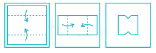Detailed Solution for RRB JE CBT 1 - Full Test 2 - Question 6

When the folded paper is opened, it appears as shown below,Thus, when the fold is opened the paper looks like image at option 1.

RRB JE CBT 1 - Full Test 2 - Question 7

As per the Central Statistic Office, the Indian economy is expected to grow at ________ in 2018-19 due to improvement in the performance of agriculture and manufacturing sectors.

Detailed Solution for RRB JE CBT 1 - Full Test 2 - Question 7

As per the Central Statistic Office, the Indian economy is expected to grow at 7.2% in 2018-19 due to improvement in the performance of agriculture and manufacturing sectors.

RRB JE CBT 1 - Full Test 2 - Question 8

Choose odd one from the given options.

Detailed Solution for RRB JE CBT 1 - Full Test 2 - Question 8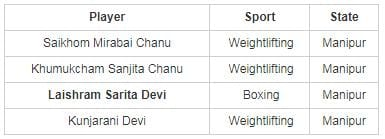RRB JE CBT 1 - Full Test 2 - Question 9

P, Q, R, S, T, W, Y, and Z are sitting around a circular table from equal distances to each other and facing towards the center. They all like different colors viz., Red, Blue, Green, Black, White, Pink, Yellow, and Brown (not necessarily in the same order). P likes yellow and sitting third to the left of W. Two persons are sitting between W and S. The one who likes pink is not sitting with P nor with W. Only one person is sitting between T and Z but not P and S. Y likes black but he is not sitting with S. R does not like pink nor white. T is sitting to the immediate left one who likes green. Z does not like brown nor he is sitting to the immediate right one who likes black. The one who likes white is not sitting with W. R neither likes brown nor Red. Which of the following combination is true as per the given information?

Detailed Solution for RRB JE CBT 1 - Full Test 2 - Question 9

Eight People: P, Q, R, S, T, W, Y and Z

Colours: Red, Blue, Green, Black, White, Pink, Yellow and Brown (not necessarily in the same order)

1) P likes yellow and sitting third to the left of W.

2) Two persons are sitting between W and S

3) The one who likes pink is not sitting with P nor with W.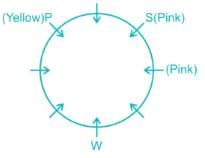4) Only one person is sitting between T and Z but not P and S.

5) Y likes black but he is not sitting with S.

6) R does not like pink nor white.

(So, R is sitting between P and S.)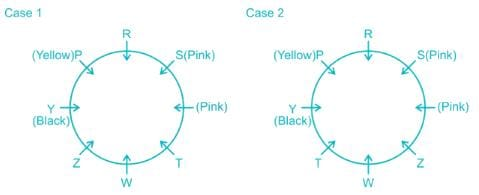7) T is sitting to the immediate left one who likes green.

(So, W likes green.)

8) Z does not like brown nor he is sitting to the immediate right one who likes black.

(Thus, case I gets eliminated.)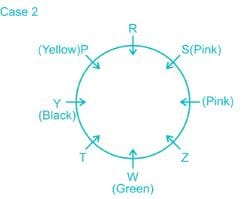9) The one who likes white is not sitting with W.

(So, S likes white (refer to point no. 7).

10) R neither likes brown nor Red.

(So, R likes blue.)

Also, Z does not like brown, so Z likes Red and T likes brown.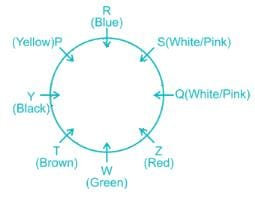Hence, “Z, Red” is the correct answer.

RRB JE CBT 1 - Full Test 2 - Question 10

In the given question, select the related word from the given alternatives.
Accrue : Diffuse :: Agony : ?

Detailed Solution for RRB JE CBT 1 - Full Test 2 - Question 10

Accrue means to accumulate or receive over time.

Diffuse means spread out over a large area.

Accrue and diffuse are antonyms to each other.

Similarly,

Agony means extreme physical or mental suffering.

Among the given words Relish is an antonym to Agony.

Relish means great enjoyment.

Thus Agony is related to Relish.

RRB JE CBT 1 - Full Test 2 - Question 11

AIveoIi is balloon-like structures within the ______.

Detailed Solution for RRB JE CBT 1 - Full Test 2 - Question 11

AIveoIi, balloon like structures are tiny air sacs within the lungs. The respiratory system comes into direct contact with the circulatory system through them. They pass carbon dioxide in the blood to the lungs while oxygen in the lungs is passed into the blood.

RRB JE CBT 1 - Full Test 2 - Question 12

Find the next number in the series.
3, 18, 3.6, 14.4, 4.8, ?

Detailed Solution for RRB JE CBT 1 - Full Test 2 - Question 12

The logic here is as follows,

3 × 6 = 18

18 ÷ 5 = 3.6

3.6 × 4 = 14.4

14.4 ÷ 3 = 4.8

4.8 × 2 = 9.6

Thus the next number in the series is 9.6.

RRB JE CBT 1 - Full Test 2 - Question 13

Where was the international kite festival kicked off on 6 January 2019?

Detailed Solution for RRB JE CBT 1 - Full Test 2 - Question 13
• In Gujarat, the nine-day long international kite festival was kicked off at Sabarmati River Front in Ahmedabad on 6 January 2019.
• The festival was a part of the celebrations for Makar Sankranti (also called Uttarayan).
• More than 150 kite fliers from 45 countries had participated in this edition of the festival.
• About 500 kite fliers from 13 different states of the country had also taken part.
RRB JE CBT 1 - Full Test 2 - Question 14

Weight of C is twice the weight of M. Weight of M is 1/9 of the weight of R. Weight of R is 5/2 of the weight of K. Weight of K is 1/3 of the weight of G. Who is the second lightest?

Detailed Solution for RRB JE CBT 1 - Full Test 2 - Question 14

Let weight of M be x.

Weight of C is twice the weight of M → C = 2x

Weight of M is 1/9 of the weight of R → M = 1/9 R → R = 9x

Weight of R is 5/2 of the weight of K → 9x = 5/2K → K = 3.6x

Weight of K is 1/3 of the weight of G → K = 1/3G → 3.6x = 1/3G → G = 10.8x

Final solution is:

G > R > K > C > M

Hence, ‘C’ is the 2nd lightest.

RRB JE CBT 1 - Full Test 2 - Question 15

The average temperature of the first three days is 27°C, and that of the next three days is 29° C, if the average of the entire week is 28.5°C, then the temperature of the last day is_

Detailed Solution for RRB JE CBT 1 - Full Test 2 - Question 15

Average = Sum of quantities/Total number of quantities

Given, average temperature of the first three days is 27°C, and that of the next three days is 29° C.

Sum of temperatures for first six days = 27 × 3 + 29 × 3 = 168° C.

Let the temperature on the last day be ‘t’.

Thus average temperature of the week =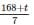Given, average of the entire week is 28.5°C.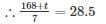⇒ 168 + t = 199.5

⇒ t = 31.5° C

RRB JE CBT 1 - Full Test 2 - Question 16

________ are the most abundant of all the cells in blood.

Detailed Solution for RRB JE CBT 1 - Full Test 2 - Question 16
• Erythrocytes are the most abundant of all the cells in the blood.
• Erythrocytes (Red Blood Cells) are found only in vertebrates and are the most abundant of all the blood cells.
• Leucocytes are the white blood cells.
• Platelets are tiny blood cells which are responsible for stopping bleeding.
• Neutrophils are the most abundant kind of white blood cells found in mammals.
RRB JE CBT 1 - Full Test 2 - Question 17

Which bank is the world's biggest financier of climate action and clean energy projects?

Detailed Solution for RRB JE CBT 1 - Full Test 2 - Question 17

European Investment Bank (EIB) will be signing a new loan for large and small clean energy projects all over India. The EIB is the world's biggest financier of climate action and clean energy projects. In 2017 the EIB invested EUR 19.6bn in climate action projects. The EIB signed a partnership with the International Solar Alliance aimed at supporting the initiative.

RRB JE CBT 1 - Full Test 2 - Question 18

If the selling price of an article is doubled, then its loss percentage gets converted into the equal profit percentage. What is the loss percentage?

Detailed Solution for RRB JE CBT 1 - Full Test 2 - Question 18

Loss % = {(Cost price – selling price)/cost price} × 100

Profit % = {(2 × selling price – cost price)/cost price} × 100

According to question,

{(Cost price – selling price)/cost price} × 100 = {(2 × selling price – cost price)/cost price} × 100

⇒ cost price – selling price = 2 × selling price – cost price

⇒ 2 × cost price = 3 × selling price

⇒ Selling price/cost price = 2/3

Now,

∴ Loss % = {(Cost price – selling price)/cost price} × 100 = {1 – (selling price/cost price)} × 100 = {1 – (2/3)} × 100 = 33.33%

Previous

RRB JE CBT 1 - Full Test 2 - Question 19

Which answer figure will complete the pattern in the question figure?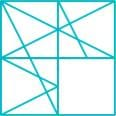Detailed Solution for RRB JE CBT 1 - Full Test 2 - Question 19

When we complete the above figure, we get: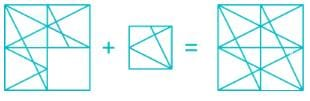RRB JE CBT 1 - Full Test 2 - Question 20

Find the next term in the series.
MATHEMATICS, ATHEMATICS, ATHEMATIC, THEMATIC, _________

Detailed Solution for RRB JE CBT 1 - Full Test 2 - Question 20

Here in each next term 1st letter form the start or 1st letter from the end is removed alternatively.

Thus the next term in the series will be THEMATI.

RRB JE CBT 1 - Full Test 2 - Question 21

In a triangle ABC, a line is drawn from C which bisects AB at point D. Find the ratio of the area of triangles DBC and ABC.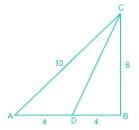Detailed Solution for RRB JE CBT 1 - Full Test 2 - Question 21

As (6, 8, 10) is a pythagorean triplet so triangle ABC is a right angled triangle, right angled at B

⇒ Area of triangle ABC = (1/2) × base × height = 1/2 × 6 × 8 = 24 cm2

Now, point D bisects AB

⇒ DB = (1/2) × AB = 1/2 × 8 = 4 cm

Also, triangle DBC is a right angle triangle, right angle at B

⇒ Area of triangle DBC = (1/2) × base × height = 1/2 × 6 × 4 = 12 cm2

∴ Required ratio = area of triangle DBC ∶ area of triangle ABC = 12 ∶ 24 = 1 ∶ 2

RRB JE CBT 1 - Full Test 2 - Question 22

Manu gives 20% of his money to his eldest son, he gives 40% of the remaining to his youngest son, he gives 30% of the remaining to a school for poor boys. Still, he has Rs. 1008. Find his total sum.

Detailed Solution for RRB JE CBT 1 - Full Test 2 - Question 22

Let’s assume that initially Manu has Rs.X

First he gives 20% of his money to his eldest son

∴ money given to the eldest son = 0.2 X

∴ Remaining money = X – 0.2 X = 0.8 X

Then he gives 40% of the remaining money to his youngest son

∴ money gives to the youngest son = 0.8 X × 0.40 = 0.32 X

∴ Remaining money = 0.8 X – 0.32 X = 0.48 X

Then he gives 30% of the remaining money to a school for poor boys

∴ money gives to school for poor boys = 0.48 X × 0.30 = 0.144 X

∴ Remaining money = 0.48 X – 0.144 X = 0.336 X

Given finally remaining money = 1008

∴ 0.336 X = 1008

⇒ X = 3000

∴ the total sum is = Rs. 3000

RRB JE CBT 1 - Full Test 2 - Question 23

According to the Indus water treaty which river will be not governed by Pakistan?

Detailed Solution for RRB JE CBT 1 - Full Test 2 - Question 23
• The Indus water treaty (IWT) is a water-distribution treaty between India and Pakistan which was signed on September 19, 1960.
• The treaty was signed by Prime Minister Jawaharlal Nehru and Pakistan’s President Ayub Khan.
• The treaty deals with river Indus and its five tributaries.
• These are divided into two categories -

Eastern Rivers:

1. Sutlej
2. Beas
3. Ravi

Western Rivers:

1. Jhelum
2. Chenab
3. Indus

According to treaty, all the water of eastern rivers shall be available for unrestricted use in India. India should let unrestricted flow of water from western rivers to Pakistan.

RRB JE CBT 1 - Full Test 2 - Question 24

Woolen clothes are used in winter season because of woollen clothes

Detailed Solution for RRB JE CBT 1 - Full Test 2 - Question 24

Woollen clothes being a bad conductor of heat does not allow body heat to dissipate in surroundings, which enables us to keep our body warm.

RRB JE CBT 1 - Full Test 2 - Question 25

Which instrument is used to measure the depth of the ocean?

Detailed Solution for RRB JE CBT 1 - Full Test 2 - Question 25

The fathom is a unit of water depth, from which the instrument gets its name. The fathometer is an echo sounding system for measurement of water depth. A fathometer will display water depth and can make an automatic permanent record of measurements.

RRB JE CBT 1 - Full Test 2 - Question 26

Directions: In making decisions about important questions, it is desirable to be able to distinguish between Strong arguments and weak arguments. Strong arguments are those, which are both important and directly related to the question. Weak arguments are those, which are of minor importance and also may not be directly related to the question or may be related to a trivial aspect of the question. The question below is followed by two arguments numbered I and II. You have to decide which of the arguments a strong argument is and which a Weak argument is.

Statement: Should English be given so much importance in India?

Argument:
I. Yes even in advanced countries the medium of instruction is English.
II. Yes. English is a much widely used language all over the world

Detailed Solution for RRB JE CBT 1 - Full Test 2 - Question 26

Clearly based on the practices in other countries a decision cannot be taken in India. Thus Argument I is invalid. Since English is used widely thus we using it in our country will help us more. Thus option 2 is the correct answer.

RRB JE CBT 1 - Full Test 2 - Question 27

Radon is formed in the alpha decay of ________.

Detailed Solution for RRB JE CBT 1 - Full Test 2 - Question 27
• The decay series begins with uranium-238, and among the substances formed is radium-226, which decays directly to radon-222.
• The uranium is widely distributed in rocks and soils throughout the earth's crust.
RRB JE CBT 1 - Full Test 2 - Question 28

A man pointed out to a woman saying that she is the wife of my maternal grandfather’s only son. What is the relation between the woman and the man’s only uncle?

Detailed Solution for RRB JE CBT 1 - Full Test 2 - Question 28

From the given information,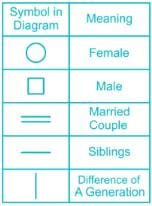Based on given data, we can draw the possible family tree.

The man in the question has only one uncle who is his maternal Grandfather’s son. The woman is the wife of the mentioned Uncle, as evident from the family tree.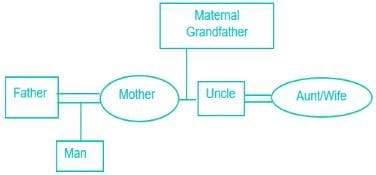Hence, that woman is Uncle’s wife.

RRB JE CBT 1 - Full Test 2 - Question 29

What would be the expenditure in 30 days at the rate of 75 paise per unit, if a bulb of 100 W is used five hours per day?

Detailed Solution for RRB JE CBT 1 - Full Test 2 - Question 29

1 unit = 1 kWh

100 W = 0.1 kW        [∵ 1kW = 1000 W]

In 30 days total consumption of the bulb = 0.1 kW × 30 days × 5 hours/day = 15 kWh

Given, cost of 1 unit = 75 paise

So total expenditure = 75 × 15 = 1125 paise = Rs. 11.25

RRB JE CBT 1 - Full Test 2 - Question 30

In the 32nd meeting of GST council held on 10 January 2019, it was decided that the companies with annual turnover up to Rs. ________ lakh will stay out of the GST net.

Detailed Solution for RRB JE CBT 1 - Full Test 2 - Question 30
• The 32nd meeting of GST council was held on 10 January 2019.
• In the meeting, it was decided that the companies with annual turnover up to Rs. 40 lakh will stay out of the GST net (Rs. 20 lakh earlier).
• The annual turnover limit for eligibility for the Composition Scheme was raised to Rs.1.5 crore from 1 April 2019.
RRB JE CBT 1 - Full Test 2 - Question 31

In the following question, select the odd image form the given alternatives.

Detailed Solution for RRB JE CBT 1 - Full Test 2 - Question 31

Here, all images except image 3 are related to physics.

i.e. image 1 is wavelength

image 2 is Reflection

image 4 is pendulum

whereas, Image 3 is structure of DNA, which is related to biology.

Hence, image 3 is odd one out.

RRB JE CBT 1 - Full Test 2 - Question 32

Identify the diagram that best represents the relationship between the given classes.
Judge, Criminal, Thief

Detailed Solution for RRB JE CBT 1 - Full Test 2 - Question 32

A ‘thief’ is a ‘criminal’. A judge is a public officer appointed to decide cases in a law court.

Hence, the relationship between Judge, Criminal, Thief can be represented as given in the below diagram: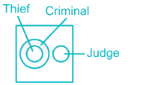Hence, option ‘2’ is the correct answer.

RRB JE CBT 1 - Full Test 2 - Question 33

In the following question, select the odd word from the given alternative.

Detailed Solution for RRB JE CBT 1 - Full Test 2 - Question 33

Here Lithium, Potassium and Rubidium are Alkali metals whereas Barium is an Alkaline earth metal.

Thus Barium is the odd word.

RRB JE CBT 1 - Full Test 2 - Question 34

A number M is divisible by 25. If (M + 5)(M + 1) is divided by 25, then what will be the remainder?

Detailed Solution for RRB JE CBT 1 - Full Test 2 - Question 34

A number M is divisible by 25 if it ends with 00, 25, 50, or 75.

(M + 5)(M + 1) = M2 + 6M + 5

When a number (x + z) is always divisible by y, the remainder of (x + z) when divided by y will be zero

When M2 + 6M + 5 is divided by 25, the remainder will be due to sum of that.

M is divisible by 25, M2 is also divisible by 25

6M will also be divisible by 25

5 when divided by 25 leaves the remainder of 5

So the sum M2 + 6M + 5 is divided by 25, the remainder will be 5

RRB JE CBT 1 - Full Test 2 - Question 35

Annual incomes of A and B are in the ratio of 5 : 3 and their annual expenses bear a ratio 3 : 1. If each of them saves Rs. 600 at the end of the year, the annual income of A is:

Detailed Solution for RRB JE CBT 1 - Full Test 2 - Question 35

Given, Annual incomes of A and B are in the ratio of 5 : 3 and their annual expenses bear a ratio 3 : 1.

Let the annual incomes of A and B be 5a and 3a respectively.

Now, each of them saves Rs. 600 at the end of the year.

Thus,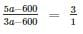⇒ 5a – 600 = 9a – 1800

⇒ 4a = 1200

⇒ a = Rs. 300

Thus, annual income of A = 5a = Rs. 1500

RRB JE CBT 1 - Full Test 2 - Question 36

Which compound released from a food processing unit causes water pollution?

Detailed Solution for RRB JE CBT 1 - Full Test 2 - Question 36

Contamination of water bodies cause water pollution, as a result of the human activities. The inorganic contaminants that cause water pollution include ammonia from food processing units, acids by industrial discharges, fertilizers, heavy metals, silt, and more.

RRB JE CBT 1 - Full Test 2 - Question 37

Directions: Study the following information carefully and answer the questions given below:

Following are the conditions for admitting a girl to a government’s girls hostel.

The girl must
i) Have a domicile certificate.
ii) Be getting some degree.
Iii) Be at least 16 years and not more than 26 years old as on 17. 01. 2016
iv) Have secured at least 50% in the last academic record.

In the case of the girl who fulfills all the conditions except ______
a) At ii) above, but not getting any degree and has scored at least 65% in the last academic record.

In this case, she should be admitted to the hostel, but has to pay 40% more fees than the others.
b) At iii) above, but from the age group, 27 to 30 years and is getting some degree. In this case, she should be admitted to the hostel, but has to pay 50% more fees than the others.

In each question below, details of one girl are provided. You have to take one of the following courses of action based on the conditions given above and the information provided in each question and mark the number of that course of action as your answer. You are not to assume anything other than the information provided in the question. All these cases are given to you as on 17. 01. 2016.

Q. Saloni Todi works at KPMG and has applied for the hostel. She has secured 90% in the last academic record and currently not getting any degree. Saloni was born on 13.04.1995 and has a domicile certificate.

Detailed Solution for RRB JE CBT 1 - Full Test 2 - Question 37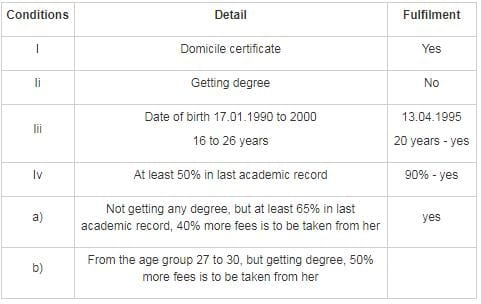Since, Saloni is fulfilling condition a), 40% more fees is to be taken from her.

RRB JE CBT 1 - Full Test 2 - Question 38

The electromagnetic wave coming out of the nucleus of a natural radioactive atom is

Detailed Solution for RRB JE CBT 1 - Full Test 2 - Question 38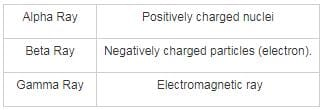RRB JE CBT 1 - Full Test 2 - Question 39

From the given alternatives find the word which can be formed from the letters used in the given word.
BARRELHOUSE

Detailed Solution for RRB JE CBT 1 - Full Test 2 - Question 39

1) ABODE → the letter D is not present in BARRELHOUSE, so it can’t be formed.

2) HERBAL → it can be formed.

3) REACH → the letter C is not present in BARRELHOUSE, so it can’t be formed.

4) LARGE → the letter G is not present in BARRELHOUSE, so it can’t be formed.

Hence, HERBAL can be formed from the letters used in BARRELHOUSE.

RRB JE CBT 1 - Full Test 2 - Question 40

The compound interest on Rs. 20480 at 25/4% p.a. for 2 years 73 days is:

Detailed Solution for RRB JE CBT 1 - Full Test 2 - Question 40

Amount after t years, when interest is compounded annually = P(1+R/100)t
Amount = 20480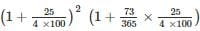= Rs.23409
C.I. = Rs. (23409 - 20480) = Rs. 2929

RRB JE CBT 1 - Full Test 2 - Question 41

If tan2y.cosec2x -1 = tan2y, then which of the following is correct -

Detailed Solution for RRB JE CBT 1 - Full Test 2 - Question 41

Given,

tan2ycosec2x - 1 = tan2y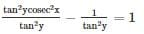We know that

cosec2x - cot2y = 1

cosec2x = 1 + cot2y

cosec2x = cosec2y

[1+ cot2θ = cosec2 θ)

∴ x = y

∴ x - y = 0

RRB JE CBT 1 - Full Test 2 - Question 42

In a certain code language if 'EXPECTED' is written as 'DPCEXETE'  then how is 'PRACTICE' written in the same code language?

Detailed Solution for RRB JE CBT 1 - Full Test 2 - Question 42

The logic here is as follows,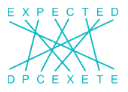Similarly,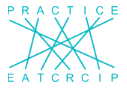Thus PRACTICE is coded as EATCRCIP.

RRB JE CBT 1 - Full Test 2 - Question 43

The number of spherical bullets that can be made out of a solid cube of lead whose edge measures 44 cm, each bullet being of 4 cm diameter, is (take π=22/7)

Detailed Solution for RRB JE CBT 1 - Full Test 2 - Question 43

Diameter of each bullet = 4 ⇒ Radius = 2 cm

Here, volume of the cube = Volume of each bullet × total no. of bullets
⇒ 44= 4/3 × 22/7 × 2× No.ofbullets
⇒ No. of bullets = 2541

RRB JE CBT 1 - Full Test 2 - Question 44

If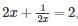then what is the value of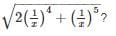Detailed Solution for RRB JE CBT 1 - Full Test 2 - Question 44

2x + 1/2x = 2 is only possible when 2x = 1

⇒ 2x = 1

⇒ x = ½

Put this in the required equation

⇒ √[2 × {1/(1/2)}4 + {1/(1/2)}5]

⇒ 23 = 8

∴ Value of required expression is 8

RRB JE CBT 1 - Full Test 2 - Question 45

If 0 ≤ x ≤ π/2, then which statement is correct -

Detailed Solution for RRB JE CBT 1 - Full Test 2 - Question 45

If 0 ≤ x ≤ π/2

Then 0 ≤ sinx ≤ 1

0 ≤ cosx ≤ 1

∴ (IV) is always right

sinx increases from 0° to 90° in first quadrant and cosx decreases.

RRB JE CBT 1 - Full Test 2 - Question 46

Find the next numbers in the series.
1, 2, 6, 12, 23, 41, ___, ____,

Detailed Solution for RRB JE CBT 1 - Full Test 2 - Question 46

The logic here is as follows,

(1 + 2) + 3 = 6

(2 + 6) + 4 = 12

(6 + 12) + 5 = 23

(12 + 23) + 6 = 41

(23 + 41) + 7 = 71

(41 + 71) + 8 = 120

Thus the next numbers in the series are 71, 120.

RRB JE CBT 1 - Full Test 2 - Question 47

In a concave mirror, if the image is formed beyond C (centre of curvature), then what will be the position of the object?

Detailed Solution for RRB JE CBT 1 - Full Test 2 - Question 47

In a concave mirror, if the image is formed beyond its centre of curvature (C), then the object must lie between C and F (focal length). The image so formed is enlarged, real and inverted.

RRB JE CBT 1 - Full Test 2 - Question 48

What is that least number that must be added to the product 684 × 686 to make it a perfect square?

Detailed Solution for RRB JE CBT 1 - Full Test 2 - Question 48

684 × 686 = 684 × (684 + 2) = 6842 + 2 × 684

To make it perfect square we should add 1

⇒ 6842 + 2 × 684 × 1 + 12 = (684 + 1)2

So ans is 1.

RRB JE CBT 1 - Full Test 2 - Question 49

A man is facing west. He turns 45 degrees in the clockwise direction and then another 180 degrees in the same direction and then 270 degrees in the anticlockwise direction. Find which direction he is facing now?

Detailed Solution for RRB JE CBT 1 - Full Test 2 - Question 49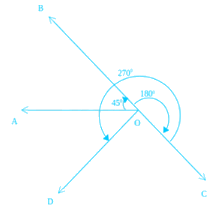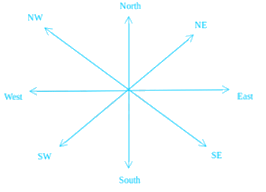The man firstly faces the direction OA. On moving 45 degrees clockwise, he faces the direction OB. Now again he moved 180 degrees clockwise, now he will be facing OC. From here he moved 270 degrees anticlockwise. Finally, he is facing OD, which is in South-west direction.

RRB JE CBT 1 - Full Test 2 - Question 50

Persons working with phosphorus develop which of the following diseases?

Detailed Solution for RRB JE CBT 1 - Full Test 2 - Question 50

Persons working with phosphorus develop a disease known as Phossy jaw in which jaw bones decay. It is also known as phosphorous necrosis of the jaw.

RRB JE CBT 1 - Full Test 2 - Question 51

In a certain code language, ‘good diet is healthy’ is written as ‘3428’, ‘diet is important’ is written as ‘821’, ‘healthy diet makes us strong’ is written as '04256. How is the word ‘important’ coded as?

Detailed Solution for RRB JE CBT 1 - Full Test 2 - Question 51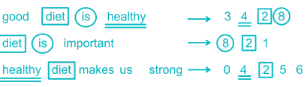Thus, ‘important’ is coded as ‘1’.

RRB JE CBT 1 - Full Test 2 - Question 52

Carbon forms which of the following bonds?

Detailed Solution for RRB JE CBT 1 - Full Test 2 - Question 52
• Carbon forms covalent bonds.
• There are three major types of covalent bonds: single, double, and triple bonds.
• A carbon atom can form four single bonds.
• A Covalent bond is a bond that is formed after the sharing of electron pairs between atoms.
RRB JE CBT 1 - Full Test 2 - Question 53

Photophobia is caused by the deficiency of ________.

Detailed Solution for RRB JE CBT 1 - Full Test 2 - Question 53

There are many causes of photophobia, bad lifestyles are the main reason. Vitamin B2 riboflavin deficiency is the main one reason why suffer photophobia.

RRB JE CBT 1 - Full Test 2 - Question 54

In a Joint family of 4 males and some female members, all members are working and the average monthly income per head is Rs.21750; while the average monthly income of male members is Rs.24000 per head and for female members, it is Rs.18750 per head. The number of female members in the family is:

Detailed Solution for RRB JE CBT 1 - Full Test 2 - Question 54

According to the given information,

Number of male members = 4

Let the number of female members be y.

We know that, Average = Sum of all observations/Number of observations

The average monthly income of male members is Rs.24000 per head

∴ 24000 = Total monthly income of males/4

⇒ Total monthly income of males = 24000 × 4 = 96000

The average monthly income of female members is Rs.18750 per head

∴ 18750 = Total monthly income of females/y

⇒ Total monthly income of females = 18750y

Now, total average monthly income = (Income of males + Income of females)/(males + females)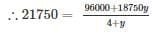⇒ 87000 + 21750y = 96000 + 18750y

⇒ 3000y = 9000

⇒ y = 3

Hence, the number of female members is 3.

RRB JE CBT 1 - Full Test 2 - Question 55

What is the value of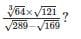Detailed Solution for RRB JE CBT 1 - Full Test 2 - Question 55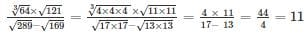RRB JE CBT 1 - Full Test 2 - Question 56

Amar started doing work, but left it after 6 days. Chetan completed the remaining work in 8 days. If Chetan alone can do the work in 24 days, how many days would Amar take to complete the remaining work?

Detailed Solution for RRB JE CBT 1 - Full Test 2 - Question 56

1 day's work of Chetan = 1/24

8 days' work of Chetan = 8 × 1/24 = 1/3

Hence, the remaining work that Chetan completed in 8 days = 1/3

⇒ Work done by Amar in 6 days = 1 – 1/3 = 2/3

⇒ 6 days' work of Amar = 2/3

⇒ 1 day's work of Amar = 1/6 × 2/3 = 1/9

This means that Amar would have done the whole work in 9 days.

∴ Amar would have taken (9 – 6 = 3) days to complete the remaining work.

RRB JE CBT 1 - Full Test 2 - Question 57

The speed of car M is twice that of car W. If car M covers 120 km in 2 hrs & 30 mins, what is the speed of car W?

Detailed Solution for RRB JE CBT 1 - Full Test 2 - Question 57

Speed of car M = (Distance Travelled)/(Time taken) = 120/2.5 = 48 kmph

∴ Speed of Car W = 48/2 = 24 kmph

RRB JE CBT 1 - Full Test 2 - Question 58

Match the following: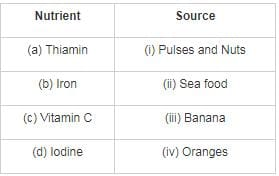Select the correct answer using the codes given below:

Detailed Solution for RRB JE CBT 1 - Full Test 2 - Question 58

Pulses and Nuts are a great source of Thiamin. Sea food gives us Iodine. Bananas have high Iron content. Oranges are a source of Vitamin C.

RRB JE CBT 1 - Full Test 2 - Question 59

If by increasing the price of a ticket in the ratio 8 : 11 the number of tickets sold fall in the ratio 23 : 21 then what is the increase (in Rs.) in revenue if revenue before the increase in the price of the ticket was Rs. 36,800?

Detailed Solution for RRB JE CBT 1 - Full Test 2 - Question 59

Let the price of the ticket be 8x and revised price be 11x (Since the ratio given is 8 ∶ 11)

Number of tickets sold be 23y and revised number be 21y,

⇒ Revenue before increase in price = 8x × 23y = 184xy

⇒ Revenue after increase in price = 11x × 21y = 231xy

Given, 184xy = 36800

⇒ xy = 200

⇒ Increased revenue = 231xy = 231 × 200 = Rs. 46200

∴ Increase in revenue = 46200 - 36800 = Rs. 9400

RRB JE CBT 1 - Full Test 2 - Question 60

As 'Car' is related to 'Door', 'Jacket' is related to

Detailed Solution for RRB JE CBT 1 - Full Test 2 - Question 60

To close the 'Car' we have a 'Door'. Similarly, Jacket has 'Zip' to close it.

Thus 'Jacket' is related to 'Zip'.

RRB JE CBT 1 - Full Test 2 - Question 61

How long will a sum of money take to double, if it is invested at 9.09% p.a. simple interest?

Detailed Solution for RRB JE CBT 1 - Full Test 2 - Question 61

SI = PRT/100, A = P + SI

SI = Simple interest, P = Principal, A = Amount, R = rate, T = time

A = 2P

2P = P + (P × 9.09 × T/100)

⇒ P = 9.09PT/100

⇒ T = 100/9.09 ≈ 11 years

RRB JE CBT 1 - Full Test 2 - Question 62

Members of which of the following have similar chemical properties?

Detailed Solution for RRB JE CBT 1 - Full Test 2 - Question 62
• Members of Isotopes have similar chemical properties.
• Isotopes of an element have the same number of electrons and protons as an atom of that element. Example: 35Cl17 and 37Cl17
• Isobars are atoms (nuclides) of different chemical elements that have the same number of nucleons.
• Isotones are the two nuclides if they have the same neutron number N, but different proton number Z.
RRB JE CBT 1 - Full Test 2 - Question 63

3 boys and 4 girls can do a piece of work in 4.5 days. Also, 9 boys and 6 girls can do the same work in 2 days. In how many days will 6 boys and 8 girls finish the same work?

Detailed Solution for RRB JE CBT 1 - Full Test 2 - Question 63

Let 1 boy’s 1 day work be ‘x’ and 1 girl’s 1 day work be ‘y’.

Hence, we have,

⇒ 3x + 4y = 1/(4.5)

⇒ 3x + 4y = 2/9           …(1)

Also,

⇒ 9x + 6y = 1/2               …(2)

Multiplying eq. (1) by 3 and subtracting from (2), we get,

⇒ 9x + 6y – 9x – 12y = 1/2– 2/3

⇒ – 6y = –1/6

⇒ y = 1/36

Substituting in (1),

⇒ x = 1/27

⇒ 6 boys and 8 girls 1 day work = 6(1/27) + 8(1/36) = 2/9 + 2/9 = 4/9

∴ 6 boys and 8 girls will finish the same piece of work in 9/4 days.

RRB JE CBT 1 - Full Test 2 - Question 64

In the given question, select the related letters from the given alternative.
AD : ZW :: GJ : ?

Detailed Solution for RRB JE CBT 1 - Full Test 2 - Question 64

In alphabetical series, the letters are related as follows,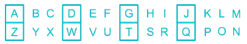Thus GJ is related to TQ.

RRB JE CBT 1 - Full Test 2 - Question 65

“Misch metal” is widely used in the manufacturing of which of the following?

Detailed Solution for RRB JE CBT 1 - Full Test 2 - Question 65

Misch metal is an alloy of rare-earth elements. Misch metal alloyed with iron is the flint (spark-producing agent) in cigarette lighters. It is also used as a deoxidizer in various alloys and to remove oxygen in vacuum tubes.

RRB JE CBT 1 - Full Test 2 - Question 66

If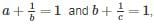then the value of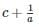is

Detailed Solution for RRB JE CBT 1 - Full Test 2 - Question 66

Given that,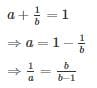and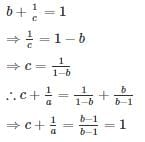RRB JE CBT 1 - Full Test 2 - Question 67

Which one of the following is a Wartime Gallantry Award?

Detailed Solution for RRB JE CBT 1 - Full Test 2 - Question 67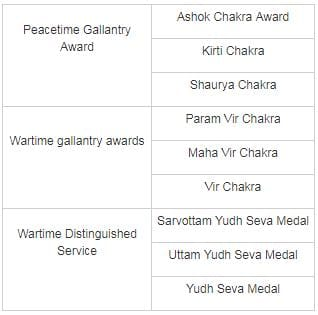RRB JE CBT 1 - Full Test 2 - Question 68

Pimples in the human body are associated with which among the following?

Detailed Solution for RRB JE CBT 1 - Full Test 2 - Question 68
• When the sebaceous glands become plugged and infected, they form skin blemishes or pimples.
• Sebaceous gland is a kind of exocrine gland which secretes sebum.
• Eccrine glands and apocrine glands are sweat glands in the human body.
• Endocrine glands are those which secrete their hormones or products directly into the blood.
RRB JE CBT 1 - Full Test 2 - Question 69

LCM of two numbers which are multiples of three is 6. The LCM of all the numbers taken together is equal to the third number. If the sum of numbers is 21, find the numbers.

Detailed Solution for RRB JE CBT 1 - Full Test 2 - Question 69

Only set of numbers that fit all the criteria is 3, 6, and 12

RRB JE CBT 1 - Full Test 2 - Question 70

Who is the author of the book titled 'We are Displaced'?

Detailed Solution for RRB JE CBT 1 - Full Test 2 - Question 70
• The book 'We are Displaced' is written by Malala Yousafzai.
• The book gives a description of her experiences of traveling around the world and visiting refugee camps.
• Malala Yousafzai got the Nobel Peace Prize in 2014.
RRB JE CBT 1 - Full Test 2 - Question 71

Which of the following bank/banks will merge with BoB to become the third largest bank in India after the State Bank of India and HDFC Bank in terms of assets?

Detailed Solution for RRB JE CBT 1 - Full Test 2 - Question 71
• On 2 January 2019, the Union Cabinet approved the merger of Vijaya Bank, Dena Bank with Bank of Baroda (BoB).
• Following the merger of these banks, BoB will become the third largest bank in India after the State Bank of India and HDFC Bank in terms of assets.
• The government of India in September 2018 had announced the merger of these three banks.
RRB JE CBT 1 - Full Test 2 - Question 72

A shopkeeper bought wheat flour at a price of Rs. 20 per kg. Using faulty weights, he gives only 900 g to a customer instead of 1 kg. If he sells the wheat flour at Rs. 25 per kg, find his profit percentage.

Detailed Solution for RRB JE CBT 1 - Full Test 2 - Question 72

Cost price of wheat flour = Rs. 20 per kg

Selling price of wheat flour = Rs. 25 per kg

Profit percentage due to price = [(25 – 20)/20] × 100 = 25%

He gives 900 g instead of 1 kg ( = 1000 g)

Profit percentage due to false weight = [error/(true weight – error)] × 100 = (100/900) × 100 = 11.1%

∴ Total profit percentage = 25 + 11.1 + (25 × 11.1)/100 = 36.1 + 2.775 = 38.875%

RRB JE CBT 1 - Full Test 2 - Question 73

Kunitha is a ritual dance form of which state?

Detailed Solution for RRB JE CBT 1 - Full Test 2 - Question 73

RRB JE CBT 1 - Full Test 2 - Question 74

On which date will the Oscars be aired in 2020?

Detailed Solution for RRB JE CBT 1 - Full Test 2 - Question 74

The Academy which runs the Oscars ceremony has added a new category to honor popular films. The Academy also plans to set an earlier air date for the Oscars in 2020 — February 9 — and will be making that broadcast more “globally accessible.” The award show will also be capped at three hours long.

Oscars 2019 -February 24

RRB JE CBT 1 - Full Test 2 - Question 75

Directions: Given are a pair of statements and conclusions. Find the conclusions that follow from the given conclusions.

Statement I: Some keys are locks, some locks are numbers.
Statement II: All numbers are letters, all letters are words.

Conclusion I: Some words are numbers
Conclusion II: Some locks are letters

Detailed Solution for RRB JE CBT 1 - Full Test 2 - Question 75

Given statements can be represented using Venn diagram as follow: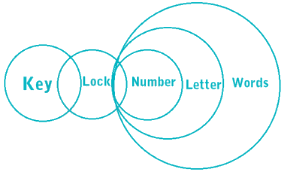Conclusion I: Some words are numbers ⇒ This is definitely true.
Conclusion II: Some locks are letters ⇒ This is definitely true.

Hence, both conclusions are true.

RRB JE CBT 1 - Full Test 2 - Question 76

Directions: In making decisions about important questions, it is desirable to be able to distinguish between Strong arguments and Weak arguments. Strong arguments are those, which are both important and directly related to the question. Weak arguments are those, which are of minor importance and also may not be directly related to the question or may be related to a trivial aspect of the question. The question below is followed by two arguments numbered I and II. You have to decide which of the arguments a strong argument is and which a Weak argument is.

Statement: Should there be only one university throughout India?

Arguments:
I. Yes. It is the only way to bring uniformity in the education system of India.
II. No. Implementing this would not be possible.

Detailed Solution for RRB JE CBT 1 - Full Test 2 - Question 76

It is not possible to have a single university in the whole country and uniformity can be achieved in other ways like following the same syllabus or pattern of questions. Thus only II holds.

RRB JE CBT 1 - Full Test 2 - Question 77

A vendor sells 40% of his stock for a particular item and recovers the cost price. If he continues to sell rest of the stock at the same price, what will be his profit percentage after selling the complete stock?

Detailed Solution for RRB JE CBT 1 - Full Test 2 - Question 77

⇒ Given: 40% of SP = 100% of CP

⇒ 1% of SP = 100% / 40% of CP

⇒ 100% of SP = 100% / 40% ⨯ 100% of CP

⇒ 100% of SP = 250% of CP

∴ Profit = SP - CP = (250 - 100)% = 150%

RRB JE CBT 1 - Full Test 2 - Question 78

Match the following items of column (A) with column (B).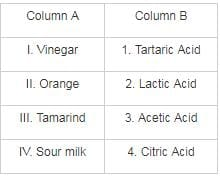Detailed Solution for RRB JE CBT 1 - Full Test 2 - Question 78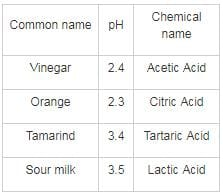RRB JE CBT 1 - Full Test 2 - Question 79

A certain bank offers 8% rate of compound interest on the 1st year and 9% on the 2nd year in a certain fixed deposit scheme. If Rs. 17,658 is received after investing for 2 years in this scheme, then what was the amount (in Rs) invested?

Detailed Solution for RRB JE CBT 1 - Full Test 2 - Question 79

According to question,

17658 = P(1 + 8/100)(1 + 9/100)

⇒ 17658 = P × 108/100 × 109/100

⇒ P = Rs. 15000

∴ The amount invested = Rs. 15000

RRB JE CBT 1 - Full Test 2 - Question 80

If it takes 20 seconds for a train to cross a 60 meters long platform, and a man standing on platform observes that it took 16 seconds for train to cross him. Find the speed of train.

Detailed Solution for RRB JE CBT 1 - Full Test 2 - Question 80

Let the length of train be x meters.
The speed of train be y m/s.

Since, Train is crossing a man, so the distance travelled by train to pass a man is equal to its own length.

Now, as we know that Speed = Distance/Time

∴ y = x/16

⇒ x = 16y

While crossing the platform total distance covered by train would be summation of length of train and length of platform.

∵ Speed = distance/time

∴ y = (x + 60)/20

⇒ 20y = x + 60

⇒ x = 20y – 60

On equating value of x, we get

16y = 20y – 60

⇒ 4y = 60

⇒ y = 15 m/s.

⇒ y =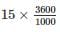kmph = 54 kmph

Speed of train is 54 kmph.

RRB JE CBT 1 - Full Test 2 - Question 81

Which of the following sequence is difference form the rest?

Detailed Solution for RRB JE CBT 1 - Full Test 2 - Question 81

The logic here is as follows,

1) 8, 20, 44, 92, 188

(2 × 2) + 4 = 8

(8 × 2) + 4 = 20

(20 × 2) + 4 = 44

(44 × 2) + 4 = 92

(92 × 2) + 4 = 188

2)  ​6, 16, 36, 76, 156

(1 × 2) + 4 = 6

(6 × 2) + 4 =16

(16 × 2) + 4 = 36

(36 × 2) + 4 = 76

(76 × 2) + 4 = 156

3) 4, 12, 28, 60, 124

(0 × 2) + 4 = 4

(4 × 2) + 4 =12

(12 × 2) + 4 = 28

(28 × 2) + 4 = 60

(60 × 2) + 4 = 124

4) 7, 22, 67, 202, 607

(2 × 3) + 1 = 7

(7 × 3) + 1 = 22

(22 × 3) + 1 = 67

(67 × 3) + 1 = 202

(202 × 3) + 1 = 607

Thus the odd series is 7, 22, 67, 202, 607.

RRB JE CBT 1 - Full Test 2 - Question 82

World Economic Forum was founded in the year ________.

Detailed Solution for RRB JE CBT 1 - Full Test 2 - Question 82

Founded in January 1971 by Klaus Schwab, the World Economic Forum is a non - profit organisation based in Switzerland. The aim of the body is to improve the state of the world by engaging business, political, academic and other leaders of society to discuss and work upon global, regional and industry issues. The forum is best known for its annual international meeting in January in Davos, a mountain resort.

RRB JE CBT 1 - Full Test 2 - Question 83

In adult mammals, Erythrocytes are formed in ________

Detailed Solution for RRB JE CBT 1 - Full Test 2 - Question 83
• Red Blood Cells or Erythrocytes of adult mammals are formed in the bone marrow of the body.
• The general lifespan of the RBC is about 120 days after which new cells replace the old ones.
RRB JE CBT 1 - Full Test 2 - Question 84

Arrange the given words in the reverse dictionary order and choose the one that comes fourth in the series.

Neutral, Nagged, Narrated, Nominate, Napoleon.

Detailed Solution for RRB JE CBT 1 - Full Test 2 - Question 84

As per the reverse dictionary order,

1) Nominate

2) Neutral

3) Narrated

4) Napoleon

5) Nagged

Hence, ‘Napoleon’ is the fourth word.

RRB JE CBT 1 - Full Test 2 - Question 85

Scissor term is associated with which of the following sport?​

Detailed Solution for RRB JE CBT 1 - Full Test 2 - Question 85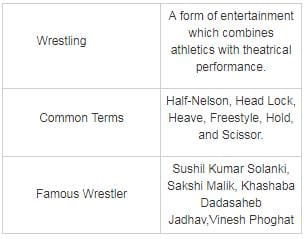RRB JE CBT 1 - Full Test 2 - Question 86

The animals in which the body cavity is absent are known as _______.

Detailed Solution for RRB JE CBT 1 - Full Test 2 - Question 86

Animals that have a body cavity called a coelom with a complete lining called peritoneum derived from mesoderm (one of the three primary tissue layers) are called Coelomates (also known as eucoelomates - "true coelom"). Contrarily, animals which lack a coelom, like flatworms and tapeworms, are called acoelomates.

RRB JE CBT 1 - Full Test 2 - Question 87

Which state's 'Katarni rice' got the Geographical Indication (GI) tag?

Detailed Solution for RRB JE CBT 1 - Full Test 2 - Question 87
• Bihar's Katarni rice has got the Geographical Indication (GI) tag.
• The Shahi litchi of North Bihar has got the Geographical Indication (GI) tag. It is the fourth product from the state after Jardalu mango, Katarni rice and Magahi Paan to have got the GI tag.
• Shahi Litchi is mainly cultivated in Muzaffarpur, Samastipur, Vaishali, East Champaran, and Begusarai and some parts of adjoining areas in agro-climatic areas of the Bihar.
RRB JE CBT 1 - Full Test 2 - Question 88

Unit of impedance is ________.

Detailed Solution for RRB JE CBT 1 - Full Test 2 - Question 88

Electrical impedance is the measure of the opposition that a circuit presents to a current when a voltage is applied. In general, impedance will be a complex number, with the same units as resistance, for which the SI unit is the ohm (Ω).

RRB JE CBT 1 - Full Test 2 - Question 89

What is the unit of the physical quantity "Stress"?

Detailed Solution for RRB JE CBT 1 - Full Test 2 - Question 89

Stress is defined as the force per unit area of a material. i.e.

Stress = force/cross sectional area: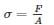where, sigma (σ)= stress, F= force applied, and A = cross sectional area of the object.

Units of stress : Nm-2 or Pa.

RRB JE CBT 1 - Full Test 2 - Question 90

Refer the below data table and answer the following question :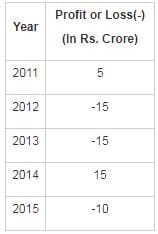Q. What was the total profit or loss of the company in the last 5 years?

Detailed Solution for RRB JE CBT 1 - Full Test 2 - Question 90

Total profit = Rs. (5 + 15) crore = Rs. 20 crore.

Total loss = Rs. (15 + 15 + 10) crore = Rs. 40 crore.

∴ The net loss = Rs. 40 - 20 crore = Rs. 20 crore.

RRB JE CBT 1 - Full Test 2 - Question 91

If 4 * 7 * 6 = 698 and 3 * 5 * 0 = 572, then 6 * 2 * 1 = ?

Detailed Solution for RRB JE CBT 1 - Full Test 2 - Question 91

In 4 * 7 * 6 = 698

The pattern followed here is,

4 + 2 = 6

7 + 2 = 9

6 + 2 = 8

In 3 * 5 * 0 = 572

3 + 2 = 5

5 + 2 = 7

0 + 2 = 2

Similarly, then adding 2 to each number 6 * 2 * 1

6 + 2 = 8

2 + 2 = 4

1 + 2 = 3

RRB JE CBT 1 - Full Test 2 - Question 92

A, B, and C are three points on a circle. The tangent AT meets the extended chord BC at T such that ∠BTA = 44°, ∠ABC = ∠CAT = 40°. The angle subtended by BC at the center of the circle is -

Detailed Solution for RRB JE CBT 1 - Full Test 2 - Question 92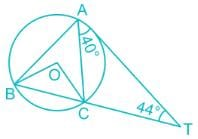⇒ Given: ∠CAT = 40° and ∠CTA = 44°

⇒ We have ∠CAT = ∠ABC = 40° (alternate segment of the circle)

⇒ Again, ∠BCA = ∠CAT + ∠CTA = 40° + 44° = 84° (exterior angle of a triangle)

⇒ Now , In ∆ABC,

⇒ ∠ABC + ∠BCA + ∠CAB = 180°

⇒ 40° + 84° + ∠CAB = 180°

⇒ ∠CAB = 56°

⇒ Again ∠BOC = 2∠CAB = 2 × 56° = 112° (angle subtended by a chord on the center is double to the angle subtended on the circle)

RRB JE CBT 1 - Full Test 2 - Question 93

The Second Ozone hole was detected over  ________.

Detailed Solution for RRB JE CBT 1 - Full Test 2 - Question 93

The Second Ozone hole was detected over the Arctic.

RRB JE CBT 1 - Full Test 2 - Question 94

The angle of depression from 100-meter tower to the top and bottom of a house is 30° and 60° respectively. What is the height of the house?

Detailed Solution for RRB JE CBT 1 - Full Test 2 - Question 94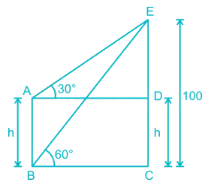Let AB = h

In ΔBCE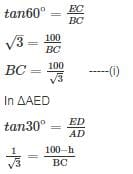BC = (100 - h) × √3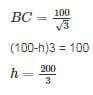RRB JE CBT 1 - Full Test 2 - Question 95

What is the unit of measurement of the Air Quality Index?

Detailed Solution for RRB JE CBT 1 - Full Test 2 - Question 95

The Air Quality Index is measured in ppm or parts per million. It is the ratio of the pollutant to a million parts of atmospheric air. An air quality index is a number used by government agencies to communicate to the public how polluted the air currently is or how polluted it is forecast to become in future.

RRB JE CBT 1 - Full Test 2 - Question 96

When the price of sugar decreased by 10%, a man could buy 1 kg more for Rs. 270. Then, the original price of sugar per kg is

Detailed Solution for RRB JE CBT 1 - Full Test 2 - Question 96

Let the original price of the sugar per kg be Rs. X. Let the quantity of sugar purchased initially be Y units in Rs. 270.

Thus, X × Y = 270 ….(1)

Given: Price of sugar decreased by 10%.

∴New price of sugar per Kg =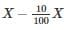= 0.9X

Since the man could buy 1 more kg on his reduction of price, hence

⇒ Quantity of sugar purchased = Y + 1

As the total expenditure remains the same i.e. Rs. 270, therefore

⇒ 0.9X × (Y + 1) = 270 …(2)

Dividing equation (1) by (2), we get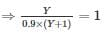⇒ Y = 0.9Y + 0.9

⇒ Y = 9 units

∴ From equation (1) we get, X = Rs. 30

RRB JE CBT 1 - Full Test 2 - Question 97

Regur Soil is also known as ________.

Detailed Solution for RRB JE CBT 1 - Full Test 2 - Question 97

Black soil is also called Regur soil. It is black in colour and ideal for growing cotton. This type of soil is typical of the Deccan trap (Basalt) region spread over North - West Deccan plateau and is made up of lava flows.

RRB JE CBT 1 - Full Test 2 - Question 98

Who among the following is a ruler in the Delhi Sultanate during whose reign there was a Mongolian Invasion in India?

Detailed Solution for RRB JE CBT 1 - Full Test 2 - Question 98
• During the reign of Masud Shah, towards the end of 1245 AD, Mongols captured Multan which was recovered by Balban.
• During the reign of Ghiyasuddin Balban, there were Mongolian invasions in 1279 AD and 1286 AD.
• During the reign of Jalaluddin Khilji, in 1292 AD, Abdullah, the leader of the Mongols, came to the northern part of India but later about 4000 Mongols were converted to Islam and came to be known as the 'New Muslaman'.
• During the reign of Firoz Shah Tughlaq, there was no Mongol Invasion but Timur, the Lame attacked India in 1398 AD i.e. after his death.
RRB JE CBT 1 - Full Test 2 - Question 99

With which of the following is 'Van Mahotsav' associated?

Detailed Solution for RRB JE CBT 1 - Full Test 2 - Question 99

Van Mahotsav is an annual tree - planting movement in India, which began in 1950. The name Van Mahotsava means Festival of forest. It was started by K.M. Munshi.

RRB JE CBT 1 - Full Test 2 - Question 100

Which is the largest lymphatic organ of the body?

Detailed Solution for RRB JE CBT 1 - Full Test 2 - Question 100
• The spleen is the largest lymphatic organ of the body.
• Lymphatic organs are the ones which carry the clear fluid lymph in them.
• The spleen synthesizes antibodies in its white pulp and removes antibody-coated bacteria.

## RRB JE (Railways Junior Engineer) CBT Mock Test Series

19 tests
 Use Code STAYHOME200 and get INR 200 additional OFF Use Coupon Code
Information about RRB JE CBT 1 - Full Test 2 Page
In this test you can find the Exam questions for RRB JE CBT 1 - Full Test 2 solved & explained in the simplest way possible. Besides giving Questions and answers for RRB JE CBT 1 - Full Test 2, EduRev gives you an ample number of Online tests for practice

19 tests